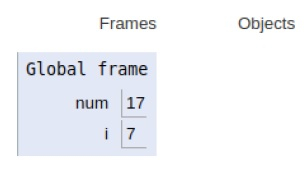# Python Program to Check Prime Number

Problem statement − We are given a number, we need to check whether the given number is a prime number or not.

A given positive number greater than 1 which has no other factors except 1 and the number itself is referred to as a prime number. 2, 3, 5, 7, etc. are prime numbers as they do not have any other factors.

In this program below, the number is checked about its prime or non-prime nature. Numbers less than or equal to 1 can not be referred to as prime numbers. Hence, we only iterate if the number is greater than 1.

Now we check whether the number is exactly divisible by any number in the range of 2 to(num - 1//2) . If any factor is found in the given range, the number is not prime. otherwise, the number is prime.

Now let’s observe the concept in the implementation below−

## Example

num = 17
if num > 1:
for i in range(2, num//2):
# If num is divisible by any number between 2 and n / 2, it is not prime
if (num % i) == 0:
print(num, "is not a prime number")
break
else:
print(num, "is a prime number")
else:
print(num, "is not a prime number")

## Output

17 is a prime numberAll the variables are declared in the local scope and their references are seen in the figure above.

## Conclusion

In this article, we have learned about the python program to check whether the given number is prime in nature or not.